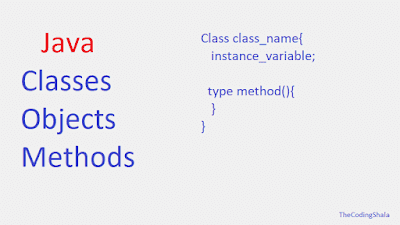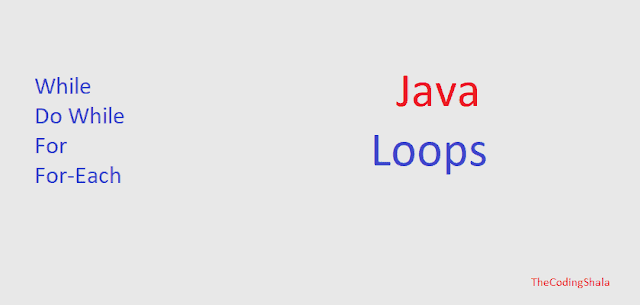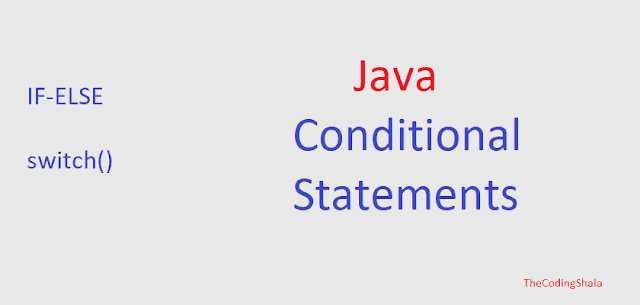## Posts

Showing posts from January, 2019

### Java Classes and Objects - The Coding ShalaHome >> Learn Java >> Java Classes and Objects Java Classes Java classes are a structure or blueprint for which we create objects. When we create a class then it defines a new data type. This new data type is used to create objects of that type.  The class keyword is used to declare a class. A class contains variables and methods. The basic structure of a class is as follows:  class class_name { type instance_variable1 ; type instance_variable2 ; ... .. type instance_variableN ; type method_name ( parameters ){ //body of method } type method_name2 ( parameters ){ //body of method } ... ... type method_nameN ( parameters ){ //body of method } } The Variables, defined within a class are called instance variables. The methods and variables defined within a class are called members of the class.  Note: Java classes do not need to have a main() method. If the class is the starting point of our program then the main() method

### Java Jump Statements - The Coding ShalaHome >> Learn Java >> Java Jump Statements Jump Statements In Java, jump statements are used to transfer control to another part of the program. There are three types of jump statements available in Java. They are break, continue, and return. Java break Statement Java break statements are basically used to exit a loop or can be used as goto statements with labels. Java break statements also used to terminate a statement sequence in a switch statement. The following Java program used the break statement to exit the loop:  class BreakExample { public static void main ( String args []){ for ( int i = 0 ; i <= 100 ; i ++){ if ( i == 10 ){ //exit loop when i is equals to 10 break ; } System . out . println ( "Value of i is: " + i ); } } } <<<<< Output of this program is >>>>> Value of i is: 0 Value of i is:

### Java Loops - The Coding ShalaHome >> Learn Java >> Java Loops Java loops In Java, loops are used to execute the same set of code again and again until termination. There are three types of loops or iteration statements are for, while, and do-while. Java while loop The basic syntax of Java while loop is as follows:  while ( condition ){ // code inside while body } The code inside the body of while loop will be executed as long as the condition is true. The condition can be any boolean expression.  The following Java program explains Java's while loop:  class WhileExample { public static void main ( String [] args ){ int num = 0 ; while ( num <= 5 ){ System . out . println ( "Value of num is: " + num ); num ++; } } } <<<<< Output of this program is >>>>> Value of num is: 0 Value of num is: 1 Value of num is: 2 Value of num is:

### Java Conditional Statements - The Coding ShalaHome >> Learn Java >> Java Conditional Statement Java's Conditional Statements Java Supports two conditional or selection statements: if and switch. These statements are used to control the flow to the program based on the condition that we have put.  Java If else Statement The basic statement of Java's If the statement is as follows:  if ( condition ){ //code } else { //code } or if ( condition1 operators condition 2 ){ //code } OR if ( codition1 ){ //code } else if ( condition2 ){ //code } else { //code } The condition is an expression returns a boolean value. The else part is optional.  Note: we can also use nested if statements. (if inside if statement) The following Java program explains the if statement:  class IfExample { public static void main ( String [] args ){ int a = 10 ; int b = 20 ; if ( a < b ){ System . out . prin

### Java Operators - The Coding ShalaHome >> Learn Java >> Java Operators Java Operators In Java, operators are used for the evaluation of expressions. There are many types of operators available in Java-like arithmetic, bitwise, relational, logical, assignment operators, etc. Arithmetic Operators To calculate mathematical expression we use arithmetic operators. The following image shows the Java arithmetic operators:  These operators are used on numeric operands and char types in Java. We cannot use them on boolean. The modulus operator, % returns the remainder of a division operation. In Java, Increment (++) and Decrement (--) operators can be used as prefix and postfix. In the Prefix form, the operand is incremented or decremented before the value is obtained for the use in the expression. In the postfix form, the previous value is used in the expression, and then the operand is modified. For example:  a = 11 ; b = ++ a ; //is similar to a = a + 1 ; b = a ;